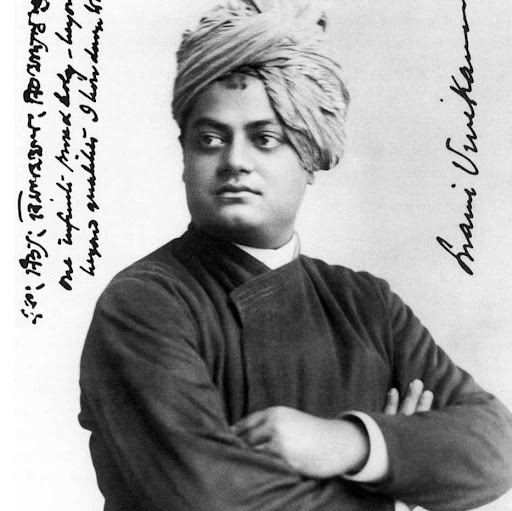6:24 AM
@yuvrajsingh hiHi sir @JohnRennieI must admit I cheated and looked at the solution, and the solution is really simple. I would probably have solved the equation of motion for the system, and it isn't necessary to go into that much detail.Sir I have doubt, can you clear that I Don, t need the full answer. @JohnRennie@yuvrajsingh yes, what's the question?So when I saw the question two things come in mt mind.6:33 AM
OK ...During the whole journey of compression and rarefaction resultant force on Centre of mass is zero, hence it move with constant velocity.
Or Centre of mass would be at rest.That certainly isn't true during the compression phase because the wall to the left of $m_1$ is exerting a force on $m_1$ so there is an external force acting.Aah, how stupid I am. So now it become more complicated.
So momentum conservation also fails only one thing left energy conservation sir. @JohnRennieExactly, yes, and energy conservation is the way you do this question.So sir what I did is.
Initial spring energy to goes to k. E of the block $m_1$,and spring and new spring energy.
@JohnRennie6:41 AM
If you compress the spring a distance $x$ then the PE is $\tfrac12 kx^2$. At this moment both masses are at rest so the KE is zero.
When the mass $m_2$ has moved back to its initial position the PE in the spring is zero so the KE of $m_2$ is now $\tfrac12 k x^2$ and the mass $m_1$ is still at rest.No sir you misunderstood me. I have three process 1,2,3 so I equate 1 directly with 3.
Ok.@JohnRennieThis is the moment the mass $m_1$ leaves the wall, and at this instant we have $m_1$ stationary and $m_2$ moving at $v = x\sqrt{k/m_2}$
And you just have to work out the velocity of the COM at this moment.Got it sir. @JohnRennie@yuvrajsingh I must admit I didn't realise it was that simple at first. I looked at the Irodov solutions :-)$v_c=x\sqrt{k/m_2}/m_1+m_2$.
Is it right sir I do not have answer.
@JohnRennieAah, answer look very simple but question doesn't, t LOL @JohnRennie:-)
To be fair Irodov often does this. The questions look hard but actually have a trick that solves them really easily.Yes my mistake I try to solve it by momentum conservation, but the haven, t thought about normal reaction force. @JohnRennie
@JohnRennie I have one more question
1.190Correct sir.7:07 AM
I'm afraid I have to leave this for now. I need to work and I've already agreed to help someone with a Windows installation.
I have the Irodov solutions manual if you want it.Yes no worry, we can discuss it later. @JohnRennie7:21 AM
@JohnRennie Do you have some time.@yuvrajsingh not for a few minutes I'm afraid. I'm working now.OK.

1 hour later…8:33 AM
@JohnRennie are you free now.

4 hours later…12:10 PM
@yuvrajsingh hi, I'm free for about half an hour if you're around.12:26 PM
Hi sir @JohnRennie@yuvrajsingh hiSo the question.
@JohnRennie same questionIf the particle moved outwards from the centre of the disk at velocity $v_0$ then their relative velocity is going to be $v = r\omega$ where $r$ is the distance from the centre of the disk. $r = v_0t$.
So in the frame of the disk the momentum of the particle will be $p = mv = mv_0t$.OkThat's tangential momentum. The radial momentum is just $mv_0$.12:34 PM
But It talk about angular momentum.The angular momentum would be $L = rp$ so just multiply by $r$ ($r = v_0 t$)
To be honest I'm nit used to working in rotating frames so while I think this is the way to go I'm making no promises!
Actually I'm afraid I've run out of time. Do you want the solution from the Irodov solutions manual?No sir I will try and think about your hint, so I can come up with my idea tommorow. @JohnRennie
Sir by the psychiatrists gave me the certificate.@yuvrajsingh good!
Hopefully you won't need it.Thanks sir. @JohnRennieSee you later :-)